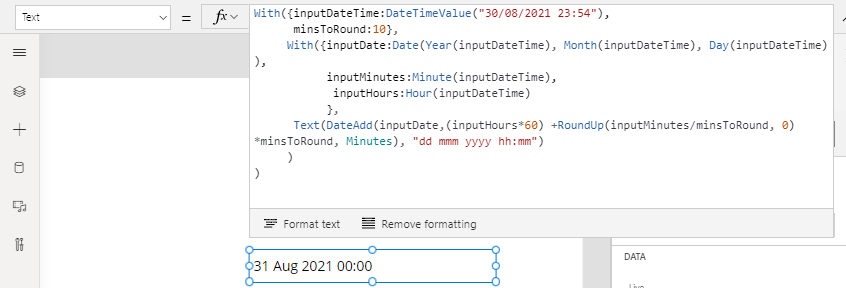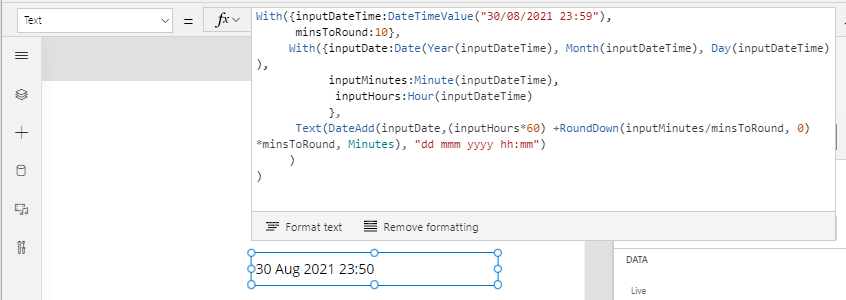# Formula - How to round times to the nearest x minutes

When working with dates and times, a common requirement is to round times up or down to the nearest X minutes. This post describes the formula to carry out this task.

For applications such as timesheet or timekeeping apps, a typical requirement is to round times up or down. The common questions and requirements from app builders include:

• How to round a datetime value to the nearest 5 minutes?
• How to round a datetime value to the nearest 10 minutes?
• How to round a datetime value to the nearest 15 minutes?
• How to round a datetime value to the nearest 30 minutes?

## Round times to the nearest X mins

The generic formula to round times is shown beneath. With this formula, we would replace inputDateTime with the datetime value that we want to round, and we would replace minsToRound with the number of minutes that we want to round to (eg 10 mins).

`With({        inputDateTime:DateTimeValue("30/08/2021 23:56"),        minsToRound:10      },      With({               inputDate:Date(Year(inputDateTime),                            Month(inputDateTime),                            Day(inputDateTime)                      ),               inputMinutes:Minute(inputDateTime),               inputHours:Hour(inputDateTime)            },            DateAdd(inputDate,                    (inputHours*60) +                        Round(inputMinutes/minsToRound, 0)*minsToRound,                    Minutes           )     ))`

The technique we apply here is to take the number of minutes (56 minutes in the above example) and to round the number of minutes to the nearest significant value that we want to round to. We apply the rounding technique that I cover in my post here:

We then take the date component of the input datetime with the time 00:00, and we add the rounded result in minutes, plus the hour component of the input datetime value in minutes. By performing this type of date arithmetic, we can successfully round the datetime value to the next day if necessary.

As an example, the screenshot beneath shows how the formula rounds "30th August 2021 23:56" to "31st August 2021 00:00" when we specify that we want to round to the nearest 10 minutes.To further verify this logic, the screenshot beneath shows how the formula rounds "30th August 2021 23:54" to "30th August 2021 23:50" when we specify that we want to round to the nearest 10 minutes.## Round UP times to the nearest X mins

Our formula relies on the Round function to round to the nearest significant value. In cases where we want to always round up to the next significant value, we would replace the call to Round with RoundUp like so.

`With({        inputDateTime:DateTimeValue("30/08/2021 23:56"),        minsToRound:10      },      With({               inputDate:Date(Year(inputDateTime),                            Month(inputDateTime),                            Day(inputDateTime)                      ),               inputMinutes:Minute(inputDateTime),               inputHours:Hour(inputDateTime)            },            DateAdd(inputDate,                    (inputHours*60) +                        RoundUp(inputMinutes/minsToRound, 0)*minsToRound,                    Minutes           )     ))`
This formula rounds up "30th August 2021 23:54" to "31st August 2021 00:00" when we specify that we want to round to the next 10 minutes, as highlighted beneath.## Round DOWN times to the nearest X mins

The natural progression of this technique is to always round down to the previous significant value. To peform this action, we would call the RoundDown function to round down the number of minutes, as shown beneath.

`With({        inputDateTime:DateTimeValue(TextInput1.Text),        minsToRound:10      },      With({               inputDate:Date(Year(inputDateTime),                            Month(inputDateTime),                            Day(inputDateTime)                      ),               inputMinutes:Minute(inputDateTime),               inputHours:Hour(inputDateTime)            },            DateAdd(inputDate,                    (inputHours*60) +                        RoundDown(inputMinutes/minsToRound, 0)*minsToRound,                    Minutes           )     ))`
This formula rounds down "30th August 2021 23:59" to "30th August 2021 23:55" when we specify that we want to round to the previous 10 minutes, as highlighted beneath.## Conclusion

This post described how to round datetime values to the nearest significant user-specified value (for example, round a time to the nearest 15 minutes). The technique is to round the minute component to the nearest significant value, and to add the result to the input datetime value (with the minute component set to 00).

For completeness, it's useful to note that we can also use string manipulation techniques to round times. The post beneath shows an example of how to do this.